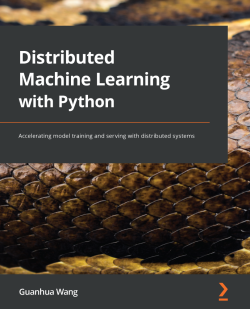# Distributed Machine Learning with Python

By Guanhua Wang
• FREE Subscription Access now
• \$39.99 Print
• \$31.99 eBook
What do you get with a Packt Subscription?

• Constantly updated with 100+ new titles each month
• Breadth and depth in over 1,000+ technologies

Reducing time cost in machine learning leads to a shorter waiting time for model training and a faster model updating cycle. Distributed machine learning enables machine learning practitioners to shorten model training and inference time by orders of magnitude. With the help of this practical guide, you'll be able to put your Python development knowledge to work to get up and running with the implementation of distributed machine learning, including multi-node machine learning systems, in no time. You'll begin by exploring how distributed systems work in the machine learning area and how distributed machine learning is applied to state-of-the-art deep learning models. As you advance, you'll see how to use distributed systems to enhance machine learning model training and serving speed. You'll also get to grips with applying data parallel and model parallel approaches before optimizing the in-parallel model training and serving pipeline in local clusters or cloud environments. By the end of this book, you'll have gained the knowledge and skills needed to build and deploy an efficient data processing pipeline for machine learning model training and inference in a distributed manner.

Publication date:
April 2022
Publisher
Packt
Pages
284
ISBN
9781801815697

# Chapter 1: Splitting Input Data

Over the recent years, data has grown drastically in size. For instance, if you take the computer vision domain as an example, datasets such as MNIST and CIFAR-10/100 consist of only 50k training images each, whereas recent datasets such as ImageNet-1k contain over 1 million training images. However, having a larger input data size leads to a much longer model training time on a single GPU/node. In the example mentioned previously, the total training time of a useable state-of-the-art single GPU training model on a CIFAR-10/100 dataset only takes a couple of hours. However, when it comes to the ImageNet-1K dataset, the training time for a GPU model will take days or even weeks.

The standard practice for speeding up the model training process is parallel execution, which is the main focus of this book. The most popular in-parallel model training is called data parallelism. In data parallel training, each GPU/node holds the full copy of a model. Then, it partitions the input data into disjoint subsets, where each GPU/node is only responsible for model training on one of the input partitions. Since each GPU only trains its local model on a subset (not the whole set) of the input data, we need to conduct a procedure called model synchronization periodically. Model synchronization is done to ensure that, after each training iteration, all the GPUs involved in this training job are on the same page. This guarantees that the model copies that are held on different GPUs have the same parameter values.

Data parallelism can also be applied at the model serving stage. Given that the fully-trained model may need to serve a large number of inference tasks, splitting the inference input data can reduce the end-to-end model serving time as well. One major difference compared to data parallel training is that in data parallel inference, all the GPUs/nodes involved in a single job do not need to communicate anymore, which means that the model synchronization phase during data parallel training is completely removed.

This chapter will discuss the bottleneck of model training with large datasets and how data parallelism mitigates this.

The following topics will be covered in this chapter:

• Single-node training is too slow
• Data parallelism – the high-level bits
• Hyperparameter tuning

# Single-node training is too slow

The vanilla model training process is to load both the training data and ML model into the same accelerator (for example, a GPU), which is called single-node training. There are mainly three steps that occur in a single node training model:

1. Input pre-processing
2. Training
3. Validation

The following diagram shows what this looks like in a typical model training workflow: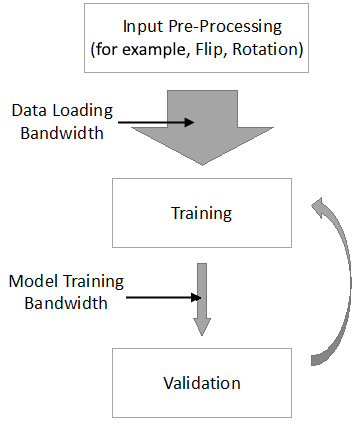Figure 1.1 – Model training workflow on a single node

As you can see, after input pre-processing, the augmented input data is loaded into the memory of the accelerators (such as GPUs). Following that, the model is trained on the loaded input data batch and validates our trained model iteratively. The goal of this section is to discuss why single-node training is way too slow. First, we will show the real bottleneck in single-node training and then describe how data parallelism mitigates this bottleneck.

Now, let's focus on the two kinds of bandwidth (BW) in this data pipeline, namely data loading bandwidth and model training bandwidth, as shown in the preceding diagram. Nowadays, we have more and more input data. Hence, we would ideally want the data loading bandwidth to be as large as possible (the wide gray arrow in the preceding diagram). However, due to the limited on-device memory of the GPUs or other accelerators, the real model training bandwidth is also limited (the narrow gray arrow in the preceding diagram).

Although it is generally believed that the larger input data size leads to a longer training time in single-node training, this is not true from the data flow perspective. From a system perspective, the mismatch between data loading bandwidth and model training bandwidth is the real issue. If we can match data loading bandwidth and model training bandwidth in single-node training, it is unnecessary to conduct in-parallel model training since distributed data processing will always introduce control overheads.

Real Bottleneck

A large input data size is not the fundamental cause of long training times in terms of single nodes. The mismatch between data loading bandwidth and model training bandwidth is the key issue.

Now that we know the reason behind the delay in single-node training when faced with large input data, let's move on to the next subtopic. Next, we will quantitively show the training times of some classic deep learning models by using standard datasets. This should help you understand why data parallel training is a must-have to deal with the mismatch between data loading bandwidth and model training bandwidth.

## Single-node training time on popular datasets

Let's directly jump into training time analysis using a single GPU. We will use an NVIDIA Tesla M60 GPU as the accelerator. First, we will train both VGG-19 and ResNet-164 on the CIFAR-10 and CIFAR-100 datasets. The following diagram shows the corresponding total training time for reaching a model test accuracy over 91%: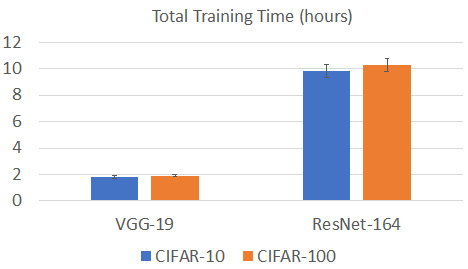Figure 1.2 – Model training time of a single node on the CIFAR-10/100 datasets

As we can see, the total training time of VGG-19 is around 2 hours for both the CIFAR-10 and CIFAR-100 datasets, while for ResNet-164, the total training time for both the CIFAR-10 and CIFAR-100 datasets is around 10 hours.

It seems that the standard model training time, when using a single GPU on the CIFAR-10/100 dataset, is neither short nor long, which is acceptable. This is mainly because of low image resolution. For the CIFAR-10/100 datasets, the resolution of each image is very low at 32x32. Thus, the intermediate results that are generated during the model training stage are relatively small, since the activation matrices in the intermediate results are always less than 32x32. Since we generate smaller activations during training in a given fixed hardware memory size, we can train more input images at once. Consequently, we can achieve a higher model training bandwidth, which mitigates the mismatch between data loading bandwidth and model training bandwidth.

Now, let's look at a modern ML model training dataset, such as ImageNet-1K. We have maintained a similar training environment setup to what we had for our CIFAR-10/100 training jobs. The difference is that we are training the VGG-19 and ResNet-50 models. The following diagram shows the corresponding total training time with a single GPU setting: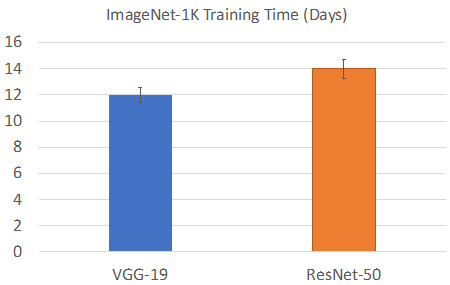Figure 1.3 – Model training time for a single node on the ImageNet-1K dataset

As we can see, the training time on a single GPU is unacceptable. It takes around 2 weeks to train a single model, such as VGG-19 or ResNet-50. The main reason for this much slower training speed on the ImageNet-1K dataset is the higher image resolution, which is now around 256x256. Having a higher image resolution means that each training image will have a bigger memory footprint for storing its activations, which means that we can only train a smaller amount of images at once. Thus, the gap between model training bandwidth and data loading bandwidth is larger. Furthermore, the training time can be even longer for wider and deeper model training.

For our machine learning practitioners, the whole model updating cycle is way too long if we only limit ourselves to using a single GPU. This long training time is amplified since we need to try multiple sets of hyperparameters and find the best training recipes.

## Accelerating the training process with data parallelism

So far, we have discussed why data parallel training is a must-have due to the mismatch between data loading bandwidth and model training bandwidth. Before we dive into the details of how data parallel training works, let's look at the speed-ups that data parallelism can achieve over single node training.

Let's take ResNet-50 training on the ImageNet-1K dataset as an example. By using a proper hyperparameter setup, the following diagram shows the normalized speedups over different GPU training baselines: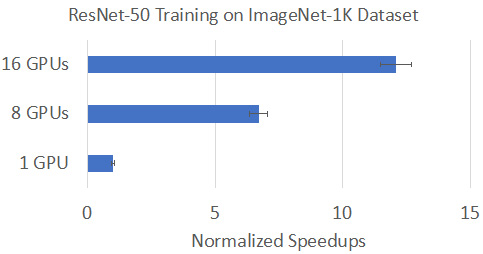Figure 1.4 – Normalized speedups over a single GPU baseline

As we can see, we have tested the system throughput for the data parallel training process over a single GPU training baseline. By incorporating multiple GPUs into the same training job, we expanded our model training bandwidth significantly with parallelism. Ideally, the extended model training bandwidth should be linearly increased by the number of GPUs involved. Due to system control overheads and network communications introduced in data parallel training, we cannot achieve linear scaling perfectly.

However, even with system overhead involved in data parallel training, the speed-up numbers are still significant compared to a single GPU training baseline. As depicted in the preceding diagram, by incorporating 8 GPUs for data parallel training, we can increase training throughput by more than 6x. With 16 GPUs involved in the same data parallel training job, the speed-up number is even better as it can achieve near 12x higher throughput compared to the single GPU baseline. Let's convert these throughput speed-up numbers into training time: if data parallel training using 16 GPUs, we can reduce ResNet-50 training on the ImageNet-1K dataset from 14 days to around just 1-2 days.

In addition, this speed-up number can continue growing when we have more GPUs involved in the same data parallel training job. With state-of-the-art hardware such as NVIDIA's DGX-1 and DGX-2 machines, the training time of ResNet-50 on the ImageNet-1K dataset can be significantly reduced to less than 1 hour if we incorporate hundreds of GPUs into this data parallel model training job.

To conclude this section, single-node model training takes up a lot of time, which is mainly due to the mismatch problem between the data loading bandwidth and the model training bandwidth. By incorporating data parallelism, we can increase the model training bandwidth proportionally to the number of accelerators involved in the same training job.

# Data parallelism – the high-level bits

So far, we have discussed the benefits of using data parallelism in machine learning model training, which can tremendously reduce the overall model training time. Now, we need to dive into some fundamental theories about how data parallel training works, such as stochastic gradient descent (SGD) and model synchronization. But before that, let's take a look at the system architecture for data parallel training, and how it is different from single-node training.

The simplified workflow for data parallel training is depicted in the following diagram. We have omitted some technical details during the training phase as we are mainly concerned with the two bandwidths (that is, the data loading bandwidth and the model training bandwidth):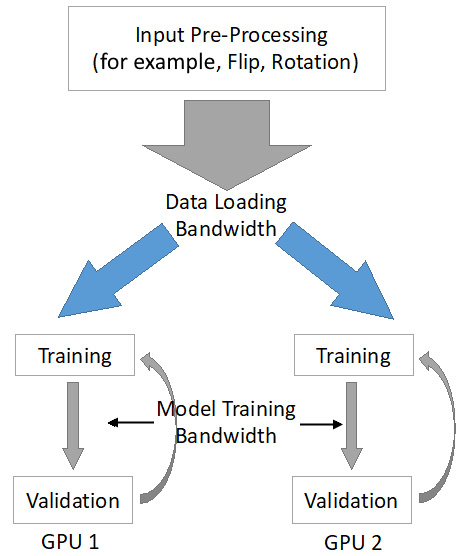Figure 1.5 – Simplified workflow of data parallel training

As we can see, the main difference between single-node training and data parallel training is that we split the data loading bandwidth between multiple workers/GPUs (shown as blue arrows in the preceding diagram). Therefore, for each GPU involved in the data parallel training job, the difference between its local data loading bandwidth and model training bandwidth is much smaller compared to the single-node case.

At a high level, even though we cannot increase the model training bandwidth on each accelerator due to hardware limitations, we can split and balance the whole data loading bandwidth across multiple accelerators. And this data loading bandwidth split is not only applicable to data parallel training. It can be directly adopted in the data parallel model serving stage.

Note

By decreasing the per-GPU data loading bandwidth, data parallel training mitigates the gap between data loading bandwidth and model training bandwidth on each GPU.

At this point, we should understand how data parallel training increases end-to-end throughput by splitting the data loading bandwidth across multiple accelerators. After each GPU receives its local batch of augmented input data, it will conduct local model training and validation. Here, model validation in data parallel training is the same as in the single-node case (there are some small variations, which we will discuss later) and we mainly focus on the difference at the training stage (excluding validation).

As shown in the following diagram, in the case of a single node, we divide the model training stage into three steps: data loading, training, and model updating. As we mentioned in the Single-node training is too slow section, data loading is for loading new mini-batches of training data. Training is done to conduct forward and backward propagations through the model. Once we've generated gradients during backward propagation, we perform the third step; that is, updating the model parameters: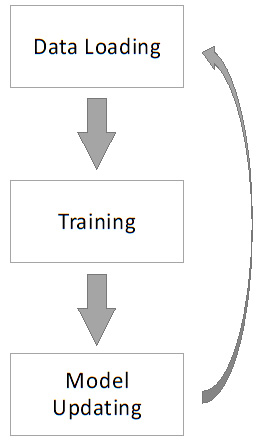Figure 1.6 – The three steps in the model training stage

Compared to the data parallel training stage, as shown in the following diagram, there are several major differences:

• First, in data parallel training, different accelerators are trained on different batches of input data (for example, Partition 1 and Partition 2 in the following diagram). Consequently, none of the GPUs can see the full training data. Thus, traditional gradient descent optimization cannot be applied here. We also need to do a stochastic approximation of gradient descent, which can be used in the single-node case. One popular stochastic approximation method is SGD. We will look at this in more detail in the next section.
• Second, in data parallel training, besides the three steps included in single-node training, as shown in the preceding diagram, we have an additional step here called model synchronization, which is shown in the following diagram. Model synchronization is about collecting and aggregating local gradients that have been generated by different nodes. We will learn more about model synchronization later in this book: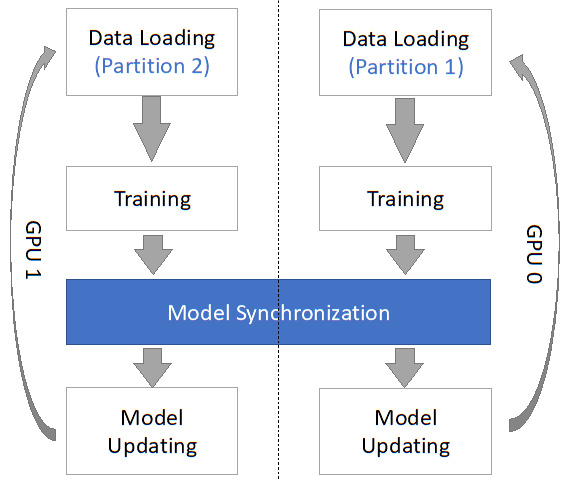Figure 1.7 – Data parallelism procedures within the model training stage

In the next two sections, we will discuss the theoretical details about SGD and model synchronization.

In this section, we will discuss why SGD is a must-have for data parallel training and how it works.

In theory, we can use traditional gradient descent (GD) for single-node training. It works as follows:

```for i in dataset:
g_all += g_i
w = w - a*g_all```

First, we need to calculate the gradients from each data point of our training dataset, where `g_i` is the gradients. Here, we calculate this on the `i`-th training data point. The formal definition of `g_i` is as follows: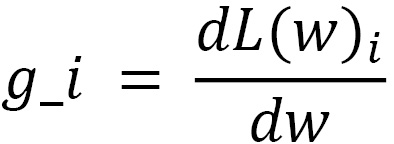Then, we sum up all the gradients that have been calculated by all the training data points (`g_all += g_i`) and then do a single step model update with `w = w - a*g_all`.

However, in data parallel training, each GPU can only see part of (not the full) training dataset, which makes it impossible to use traditional GD optimization since we cannot calculate `g_all` in this case. Thus, SGD is a must-have. In addition, SGD is also applicable to single-node training. SGD works as follows:

```for i in dataset:
w = w - a*g_i```

Basically, instead of updating the model weights (w) after generating the gradients from all the training data, SGD allows for model weights updates using a single or a few training samples (for example, a mini-batch). With this relaxation of model updating restrictions, the workers in data parallel training can update their model weights using their local (not global) training samples.

GD versus SGD

In GD, we need to compute the gradients over all the training data and update the model weights.

In SGD, we compute the gradients over a subset of all the training data and update the model weights.

However, since each worker updates their model weights based on their local training data, the model parameters of different workers can be different after each of the training iterations. Therefore, we need to conduct model synchronization periodically to guarantee that all the workers are on the same page, meaning that they maintain the model parameters after each training iteration.

## Model synchronization

As we saw previously, in data parallel training, different workers train their local models using disjointed subsets of the total training data, so the trained model weights may be different. To force all the workers to have the same view of the model parameters, we need to conduct model synchronization.

Let's study this in a simple four-GPU setting, as shown in the following diagram: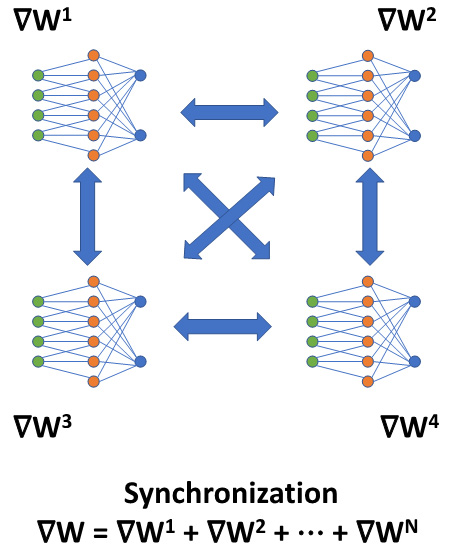Figure 1.8 – Model synchronization in a four-GPU setting

As we can see, we have four GPUs in a data parallel training job. Here, each GPU maintains a copy of the full ML model locally inside its on-device memory.

Let's assume that all the GPUs are initialized with the same model parameters, which is a standard practice, by setting the randomize function with a fixed seed.

After the first training iteration, each GPU will generate its local gradients as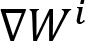, where i refers to the i-th GPU. Given that they are training on different local training inputs, all the gradients from different GPUs may be different. To guarantee that all four GPUs have the same model updates, we need to conduct model synchronization before the model parameter updates:Model synchronization does two things:

1. Collects and sums up all the gradients from all the GPUs in use, as shown here: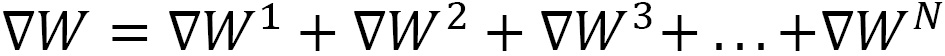Once the model synchronization steps have been completed, we can get the aggregated gradients,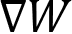, locally on each GPU. Then, we can use these aggregated gradients,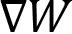, for the model updates, which guarantees that the updated model parameters remain the same after this first data parallel training iteration.

Similarly, in the following training iterations, we conduct model synchronization after each GPU generates its local gradients. So, model synchronization guarantees that the model parameters remain the same after every training iteration in a particular data parallel training job.

For the real system implementations, this model synchronization mainly has two different variations: the parameter server architecture and the All-Reduce architecture, which we will discuss in detail in the next chapter.

So far, we have come across some of the key concepts in data parallel training jobs, such as SGD and model synchronization. Next, we will discuss some important hyperparameters related to data parallel training.

# Hyperparameter tuning

In this section, we will focus on the hyperparameters that are closely related to data parallel training: global batch size, learning rate adjustment, and optimizer selection.

Let's discuss them one by one.

Notes on Hyperparameters

While some of these hyperparameters have existed in the standard single-node training process, in data parallel training, these parameters may have new searching dimensions and new correlations.

## Global batch size

The global batch size refers to how many training samples will be loaded into all the GPUs for training simultaneously. The counterpart of this concept in single-node training is the batch size or mini-batch.

Selecting the proper global batch size is different from selecting a single node's batch size. In single-node training, we always set the batch size to be the maximum number that can fit into the accelerator's memory without causing out-of-memory (OOM) issues. In data parallel training, given N GPUs, we may not set the global batch-size to be N*Max(single_node), where Max(single_node) refers to the maximum batch size on a single GPU.

In data parallel training, this global batch size is the first hyperparameter we need to search or fine-tune. If the global batch size is too large, the training model may not converge. If the global batch size is too small, it is just a waste of distributed computational resources.

Since we have used a very large global batch size compared to single node training, we also need to adjust the learning rate accordingly.

Rule of Thumb Regarding Learning Rate Adjustment

The rule of thumb policy for determining the learning rate in data parallel training is to multiply the learning rate in the single-node case by N, if we use N GPUs to do the data parallel training together.

Recent research literature suggests that, for large-batch data parallel training, we should have a warmup stage at the very beginning of the training stage. This warmup policy suggests that we should start data parallel training with a relatively small learning rate. After this warmup period, we should gradually increase the learning rate for several epochs of training, and then stop increasing the learning rate by defining a peak learning rate.

## Model synchronization schemes

Now that we have chosen our optimizer (global batch size) and adjusted the learning rate accordingly, the next thing we need to do is select an appropriate model synchronization model to use. We need this because we need to initialize a group of processes to run our data parallel training job in a distributed manner, where each process will be responsible for handling model synchronization on one machine or one GPU.

Let's take `pytorch` as an example. Here, you need to initialize your process groups, as follows:

```torch.distributed.init_process_group(backend='nccl',
init_method = '...',
world_size = N,
timeout = M)
```

Here, the first parameter (`backend='nccl'`) we need to choose from is the model synchronization backend. Right now, deep learning platforms such as PyTorch mainly support three different communication backends: NCCL, Gloo, and MPI.

The main differences among these three communication backends are as follows:

• NCCL:
• GPU only
• No support for one-to-all communication primitives such as Scatter
• No support for all-to-one communication primitives such as Gather
• Gloo:
• Mainly support for CPU, partial support for GPU.
• For CPU, it supports most communication primitives.
• For GPU, it only supports the most commonly used communication primitives, such as Broadcast and All-Reduce.
• No support for all-to-all communication.
• MPI:
• CPU only
• Supports special hardware communication, such as IP over InfiniBand

Among these three, the following are some high-level suggestions on selecting communication schemes:

• For GPU clusters, use NCCL.
• For CPU clusters, use Gloo first. If that doesn't work, try MPI.

With that, we have discussed three main communication schemes we can use in data parallel training jobs. Since the nodes we have used for model training are GPUs, we usually set NCCL as our default communication backend.

# Summary

After reading this chapter, you should be able to explore and find the real bottleneck in single-node training. You should also know how data parallelism mitigates this bottleneck in single-node training, thus increasing the overall throughput. Finally, you should know about the several main hyperparameters related to data parallel training.

In the next chapter, we will focus on two major system architectures for data parallel training, namely the parameter server (PS) and All-Reduce paradigms.